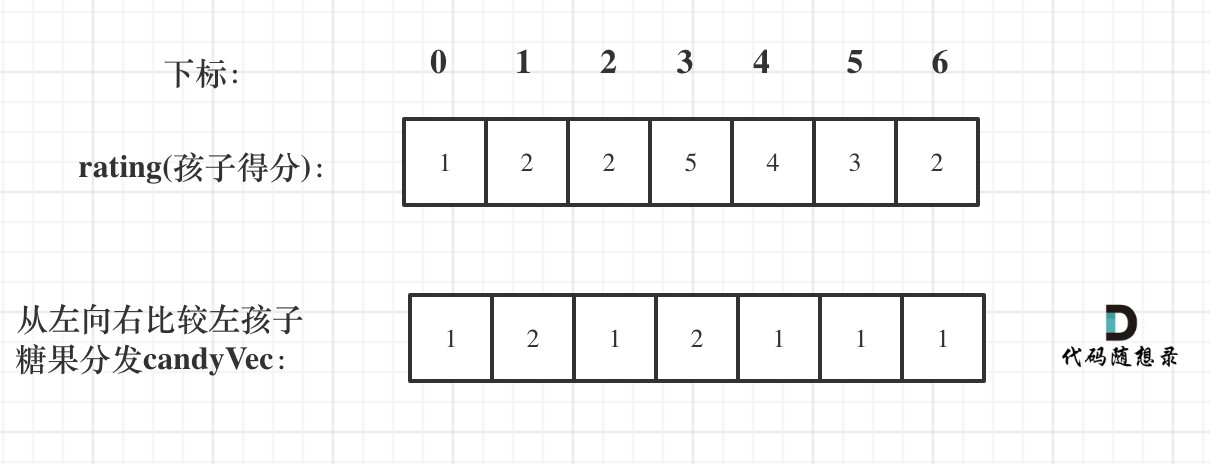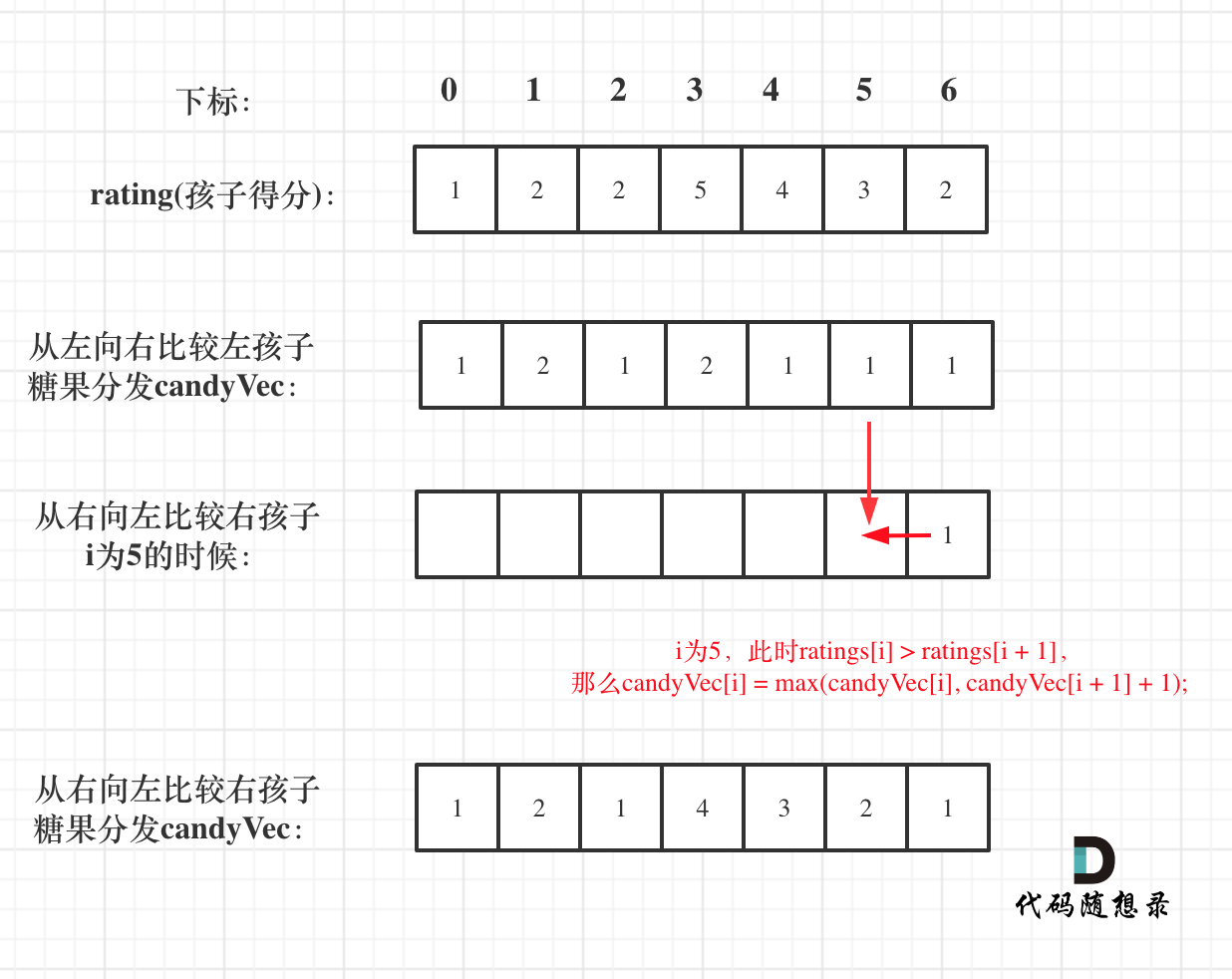# 135. 分发糖果

• 每个孩子至少分配到 1 个糖果。
• 相邻的孩子中，评分高的孩子必须获得更多的糖果。

• 输入: [1,0,2]
• 输出: 5
• 解释: 你可以分别给这三个孩子分发 2、1、2 颗糖果。

• 输入: [1,2,2]
• 输出: 4
• 解释: 你可以分别给这三个孩子分发 1、2、1 颗糖果。第三个孩子只得到 1 颗糖果，这已满足上述两个条件。

# 思路

// 从前向后
for (int i = 1; i < ratings.size(); i++) {
if (ratings[i] > ratings[i - 1]) candyVec[i] = candyVec[i - 1] + 1;
}
1
2
3
4// 从后向前
for (int i = ratings.size() - 2; i >= 0; i--) {
if (ratings[i] > ratings[i + 1] ) {
candyVec[i] = max(candyVec[i], candyVec[i + 1] + 1);
}
}
1
2
3
4
5
6

class Solution {
public:
int candy(vector<int>& ratings) {
vector<int> candyVec(ratings.size(), 1);
// 从前向后
for (int i = 1; i < ratings.size(); i++) {
if (ratings[i] > ratings[i - 1]) candyVec[i] = candyVec[i - 1] + 1;
}
// 从后向前
for (int i = ratings.size() - 2; i >= 0; i--) {
if (ratings[i] > ratings[i + 1] ) {
candyVec[i] = max(candyVec[i], candyVec[i + 1] + 1);
}
}
// 统计结果
int result = 0;
for (int i = 0; i < candyVec.size(); i++) result += candyVec[i];
return result;
}
};
1
2
3
4
5
6
7
8
9
10
11
12
13
14
15
16
17
18
19
20

# 总结

• 一次是从左到右遍历，只比较右边孩子评分比左边大的情况。
• 一次是从右到左遍历，只比较左边孩子评分比右边大的情况。

# 其他语言版本

# Java

class Solution {
/**
分两个阶段
1、起点下标1 从左往右，只要 右边 比 左边 大，右边的糖果=左边 + 1
2、起点下标 ratings.length - 2 从右往左， 只要左边 比 右边 大，此时 左边的糖果应该 取本身的糖果数（符合比它左边大） 和 右边糖果数 + 1 二者的最大值，这样才符合 它比它左边的大，也比它右边大
*/
public int candy(int[] ratings) {
int[] candyVec = new int[ratings.length];
candyVec = 1;
for (int i = 1; i < ratings.length; i++) {
if (ratings[i] > ratings[i - 1]) {
candyVec[i] = candyVec[i - 1] + 1;
} else {
candyVec[i] = 1;
}
}

for (int i = ratings.length - 2; i >= 0; i--) {
if (ratings[i] > ratings[i + 1]) {
candyVec[i] = Math.max(candyVec[i], candyVec[i + 1] + 1);
}
}

int ans = 0;
for (int s : candyVec) {
ans += s;
}
return ans;
}
}
1
2
3
4
5
6
7
8
9
10
11
12
13
14
15
16
17
18
19
20
21
22
23
24
25
26
27
28
29
30

# Python

class Solution:
def candy(self, ratings: List[int]) -> int:
candyVec =  * len(ratings)
for i in range(1, len(ratings)):
if ratings[i] > ratings[i - 1]:
candyVec[i] = candyVec[i - 1] + 1
for j in range(len(ratings) - 2, -1, -1):
if ratings[j] > ratings[j + 1]:
candyVec[j] = max(candyVec[j], candyVec[j + 1] + 1)
return sum(candyVec)
1
2
3
4
5
6
7
8
9
10

# Go

func candy(ratings []int) int {
/**先确定一边，再确定另外一边
1.先从左到右，当右边的大于左边的就加1
2.再从右到左，当左边的大于右边的就再加1
**/
need:=make([]int,len(ratings))
sum:=0
//初始化(每个人至少一个糖果)
for i:=0;i<len(ratings);i++{
need[i]=1
}
//1.先从左到右，当右边的大于左边的就加1
for i:=0;i<len(ratings)-1;i++{
if ratings[i]<ratings[i+1]{
need[i+1]=need[i]+1
}
}
//2.再从右到左，当左边的大于右边的就右边加1，但要花费糖果最少，所以需要做下判断
for i:=len(ratings)-1;i>0;i--{
if ratings[i-1]>ratings[i]{
need[i-1]=findMax(need[i-1],need[i]+1)
}
}
//计算总共糖果
for i:=0;i<len(ratings);i++{
sum+=need[i]
}
return sum
}
func findMax(num1 int ,num2 int) int{
if num1>num2{
return num1
}
return num2
}
1
2
3
4
5
6
7
8
9
10
11
12
13
14
15
16
17
18
19
20
21
22
23
24
25
26
27
28
29
30
31
32
33
34
35

# Javascript:

var candy = function(ratings) {
let candys = new Array(ratings.length).fill(1)

for(let i = 1; i < ratings.length; i++) {
if(ratings[i] > ratings[i - 1]) {
candys[i] = candys[i - 1] + 1
}
}

for(let i = ratings.length - 2; i >= 0; i--) {
if(ratings[i] > ratings[i + 1]) {
candys[i] = Math.max(candys[i], candys[i + 1] + 1)
}
}

let count = candys.reduce((a, b) => {
return a + b
})

return count
};
1
2
3
4
5
6
7
8
9
10
11
12
13
14
15
16
17
18
19
20
21@2021-2022 代码随想录 版权所有 粤ICP备19156078号-3